# Figures and data tables for SPM Figure 3a of emission pathways¶

## Notebook spm_sr15_figure_3a_global_emissions_pathways¶

This notebook is based on the Release 1.1 of the IAMC 1.5C Scenario Explorer and Data and refers to the published version of the IPCC Special Report on Global Warming of 1.5C (SR15).

The notebook is run with pyam release 0.5.0.

The source code of this notebook is available on GitHub (release 2.0.2).

spm_sr15_figure_3a_global_emissions_pathways

### IPCC SR15 scenario assessment¶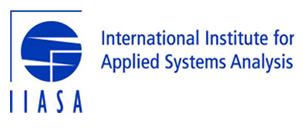# Global emission pathway characteristics¶

## Figure 3a of the Summary for Policymakers¶

This notebook extracts the emissions pathways for Figure 3a in the Summary for Policymakers of the IPCC's "Special Report on Global Warming of 1.5°C".

The scenario data used in this analysis can be accessed and downloaded at https://data.ene.iiasa.ac.at/iamc-1.5c-explorer.

### Disclaimer¶

The figures shown in this notebook are NOT the same figures as used in Figure 3a of the SPM. They are simplified figures included here only for reference.

## Load pyam package and other dependencies¶

In :
import pandas as pd
import numpy as np
import io
import itertools
import yaml
import math
import matplotlib.pyplot as plt
plt.style.use('style_sr15.mplstyle')
%matplotlib inline
import pyam

pyam - INFO: Running in a notebook, setting pyam logging level to logging.INFO and adding stderr handler


## Import scenario data, categorization and specifications files¶

Alternatively, it can be re-created using the notebook sr15_2.0_categories_indicators.

The last cell of this section loads and assigns a number of auxiliary lists as defined in the categorization notebook.

In :
sr1p5 = pyam.IamDataFrame(data='../data/iamc15_scenario_data_world_r2.0.xlsx')

pyam.utils - INFO: Reading ../data/iamc15_scenario_data_world_r2.0.xlsx

In :
sr1p5.load_meta('sr15_metadata_indicators.xlsx')

pyam.core - INFO: Importing metadata for 416 scenarios (for total of 416)

In :
with open("sr15_specs.yaml", 'r') as stream:

rc = pyam.run_control()
for item in specs.pop('run_control').items():
rc.update({item: item})
cats = specs.pop('cats')
cats_15 = specs.pop('cats_15')
cats_15_no_lo = specs.pop('cats_15_no_lo')
marker= specs.pop('marker')


## Downselect scenario ensemble to categories of interest for this assessment¶

This figure only includes scenarios where the 2010 Kyoto GHG emissions are in line with the valid range as determined by the Second Assessment Report.

In :
sr1p5.meta.rename(columns={'Kyoto-GHG|2010 (SAR)': 'kyoto_ghg_2010'}, inplace=True)

In :
df = sr1p5.filter(category=cats_15, kyoto_ghg_2010='in range')

In :
df.set_meta(meta='1.5C limited overshoot', name='supercategory', index=df.filter(category=cats_15_no_lo))
rc.update({'color': {'supercategory': {'1.5C limited overshoot': 'xkcd:bluish'}}})


## Set specifications for filters and initialize data list¶

In :
filter_args = dict(df=df, category=cats, marker=None, join_meta=True)

In :
data = []


## Plot different emissions pathways by category¶

### Net carbon dioxide emissions for all pathways limiting global warming to 1.5°C by the end of the century¶

In :
co2 = (
df.filter(variable='Emissions|CO2')
.convert_unit('Mt CO2/yr', 'Gt CO2/yr')
)
data.append(('Net carbon dioxide', co2))

In :
_co2 = co2.filter(category=cats_15, year=range(2010, 2101, 5))

fig, ax = plt.subplots()
_co2.filter(year=).line_plot(ax=ax, color='category', linewidth=2)
_co2.line_plot(ax=ax, color='category', linewidth=0.1, fill_between=True, final_ranges=True)
_co2.filter(marker=marker).line_plot(ax=ax, color='category')

Out:
<matplotlib.axes._subplots.AxesSubplot at 0x1a3511b8d0>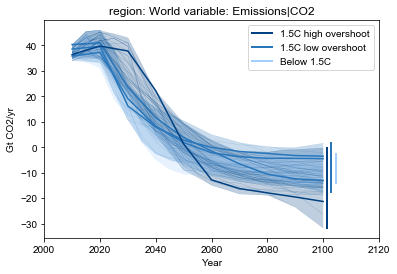### Emissions of methane, black carbon and nitrous oxide for 1.5°C pathways with limited overshoot¶

The figures below are shown as reduction relative to 2010.

In :
def plot_relative(data, baseyear=2010):
_data = data.timeseries()
_data_rel = pd.DataFrame()
for y in range(2010, 2101, 5):
_data_rel[y] = _data[y] / _data

_data_rel.reset_index(inplace=True)
_data_rel['unit'] = 'relative to {}'.format(baseyear)
_df = pyam.IamDataFrame(_data_rel)
_df.set_meta(meta='1.5C limited overshoot', name='supercategory')
_df.filter(supercategory='1.5C limited overshoot', year=range(2010, 2101, 5))\
.line_plot(color='supercategory', linewidth=0.1, fill_between=True, legend=False)

In :
ch4 = df.filter(variable='Emissions|CH4')
data.append(('Methane', ch4))

In :
plot_relative(ch4)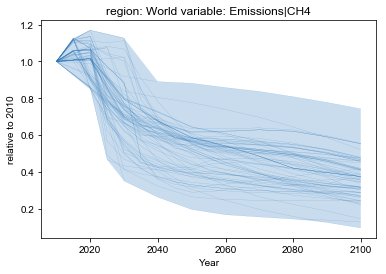In :
bc = df.filter(variable='Emissions|BC')
data.append(('Black carbon', bc))

In :
plot_relative(bc)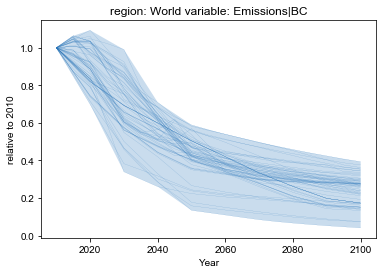In :
n2o = df.filter(variable='Emissions|N2O')
n2o.convert_unit('kt N2O/yr', 'Mt N2O/yr', factor=1/1000, inplace=True)
data.append(('Nitrous oxide', n2o))

In :
plot_relative(n2o)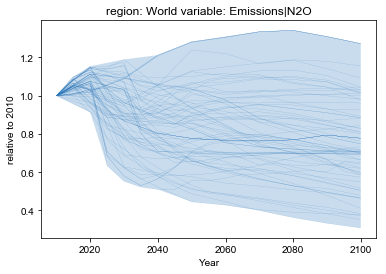## Save timeseries data to xlsx¶

In :
writer = pd.ExcelWriter('output/spm_sr15_figure3a_data_table.xlsx')
for (name, _df) in data:
pyam.utils.write_sheet(writer, name,
pyam.filter_by_meta(_df.timeseries(), **filter_args), index=True)
writer.save()

In [ ]: